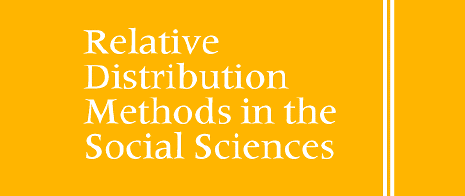# The reldist package

This is an R package to implement relative distribution methods. These methods are described in the book Relative Distribution Methods in the Social Sciences, by Mark S. Handcock and Martina Morris, Springer-Verlag, Inc., New York, 1999 ISBN 0387987789 .

It was developed by Mark S. Handcock.

It is software to estimate and graph the relative density, cumulative distribution function (CDF) and related functions. Also functions to estimate summary measures and their uncertainties. This contains functions directly related to the relative distribution and some data used in the book.

## Installation

To install the latest development version from github, the best way it to use git to create a local copy and install it as usual from there. If you just want to install it, you can also use:

``````# If devtools is not installed:
# install.packages("devtools")

devtools::install_github("handcock/reldist")``````

## Resources

• For a more detailed description of what can be done with the `reldist` package, see the introductory vignette by Handcock & Aldrich (2002) obtained at SSRN: http://dx.doi.org/10.2139/ssrn.1515775

To run an example use:

``library(reldist)``

``data(nls, package="reldist")``

A simple example comparing permanent wages of the original to the recent cohort in the NLS. See H&M (1999) for details.

``reldist(y=recent\$chpermwage,yo=original\$chpermwage,method="bgk")``

A more sophisticated version of the same.

``````reldist(y=recent\$chpermwage, yo=original\$chpermwage,
yowgt=original\$wgt, ywgt=recent\$wgt,
bar=TRUE,
smooth=0.1, method="bgk",
yolabs=seq(-1, 3, by=0.5),
ylim=c(0, 3.0),cex=0.8,
ylab="Relative Density",
xlab="Proportion of the Original Cohort")``````

A CDF version.

``````reldist(y=recent\$chpermwage, yo=original\$chpermwage,
yowgt=original\$wgt, ywgt=recent\$wgt,
cdfplot=TRUE,
smooth=0.4,
yolabs=seq(-1,3,by=0.5),
ylabs=seq(-1,3,by=0.5),
cex=0.8,
method="bgk",
ylab="proportion of the recent cohort",
xlab="proportion of the original cohort")``````